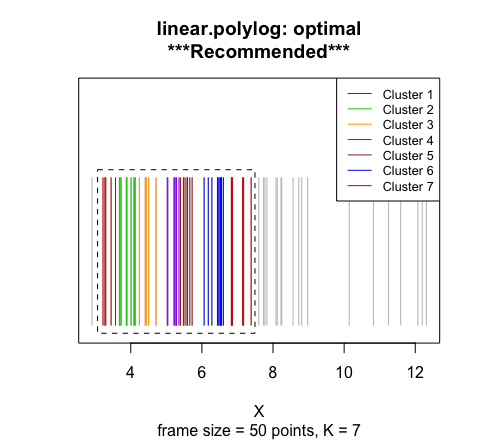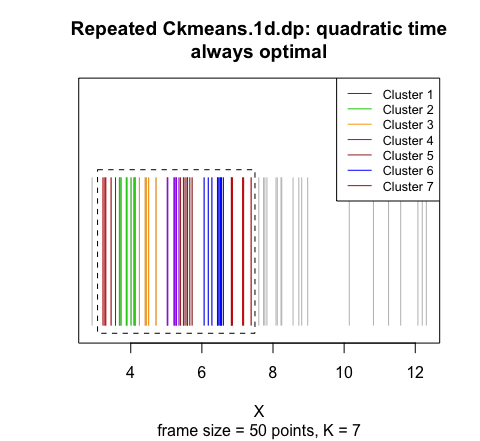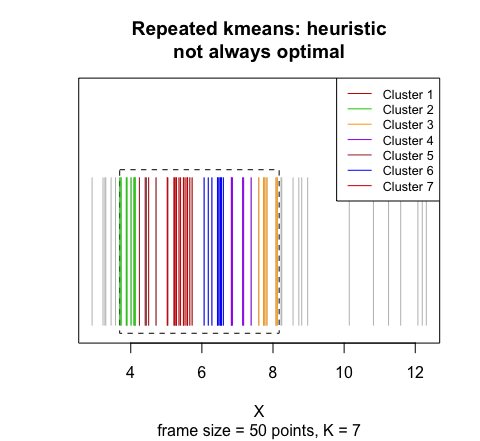# Tutorial on optimal framed clustering

#### Updated: 2021-07-27; 2020-12-12; Created: 2020-12-03

The FramedClust function (Debnath and Song 2021) finds a frame of consecutive points belonging to a large set of univariate data points to obtain the best clustering. The frame.size parameter indicates the number of points to be included in each frame. The actual width of a frame is the distance between the smallest and largest data points inside the frame.

Here we illustrate how to run the FramedClust function and visualize the results to explain what framed clustering is.

## Data preparation

Any linear dataset can be used with the FramedClust function. The input vector X contains some points from a linear data. X does not have to be sorted. We will find the best K=7 clusters among all possible frames containing 50 points (frame.size = 50).

library(OptCirClust)
X = rgamma(70, 6)
K = 7
frame.size = 50

## Performing framed clustering on the data

Now we demonstrate how to cluster the data using three different algorithms: the recommended fast and optimal "linear.polylog" algorithm, the brute-force and optimal algorithm by repeatedly calling "Ckmeans.1d.dp", and the slow and heuristic algorithm by repeatedly calling "kmeans".

# Our recommended method is the fast and optimal linear.polylog:
result_linear.polylog <- FramedClust(X, K, frame.size,  method = "linear.polylog")

# The slow and optimal via repeatedly calling Ckmeans.1d.dp:
result_Ckmeans.1d.dp <- FramedClust(X, K, frame.size,  method = "Ckmeans.1d.dp")

# The slow and heuristic via repeatedly calling kmeans:
result_kmeans <- FramedClust(X, K, frame.size, method = "kmeans")

## Visualizing framed clusters

The clustering outcomes obtained from the FramedClust function can be visualized using the plot function.

plot(result_linear.polylog, main = "linear.polylog: optimal\n***Recommended***")plot(result_Ckmeans.1d.dp, main = "Repeated Ckmeans.1d.dp: quadratic time\nalways optimal")plot(result_kmeans, main = "Repeated kmeans: heuristic\nnot always optimal")The points are colored according to their cluster. The black dashed line represents an optimal frame with the minimum sum of squared within-cluster distances. All points outside the frame are colored in gray. The "kmeans" option of the FramedClust function does not guarantee an optimal solution, while the other two method options do.